### MATRIX Statement

MATRIX M_name pattern_definitions ;

The MATRIX statement defines a matrix
and the vector, which can be given in the MINQUAD or MAXQUAD statement. The matrixand vectorare initialized to zero, so that only the nonzero elements are given. The five different forms of the MATRIX statement are illustrated with the following example: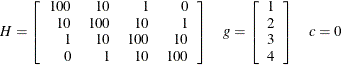Each MATRIX statement first names the matrix or vector and then lists its elements. If more than one MATRIX statement is given for the same matrix, the later definitions override the earlier ones.

The rows and columns in matrixand vectorcorrespond to the order of decision variables in the DECVAR statement.

• Full Matrix Definition: The MATRIX statement consists of H_name or g_name followed by an equals sign and all (nonredundant) numerical values of the matrixor vector. Assuming symmetry, only the elements of the lower triangular part of the matrixmust be listed. This specification should be used mainly for small problems with almost densematrices.

   MATRIX H=  100
10 100
1  10 100
0   1  10 100;
MATRIX G= 1  2  3  4;

• Band-diagonal Matrix Definition: This form of pattern definition is useful if thematrix has (almost) constant band-diagonal structure. The MATRIX statement consists of H_name followed by empty brackets
, an equals sign, and a list of numbers to be assigned to the diagonal and successive subdiagonals.

   MATRIX H[,]=  100 10 1;
MATRIX G= 1  2  3  4;

• Sparse Matrix Definitions: In each of the following three specification types, the H_name or g_name is followed by a list of pattern definitions separated by commas. Each pattern definition consists of a location specification in brackets on the left side of an equals sign that is followed by a list of numbers.

• (Sub)Diagonalwise: This form of pattern definition is useful if thematrix contains nonzero elements along diagonals or subdiagonals. The starting location is specified by an index pair in brackets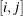. The expression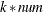on the right-hand side specifies thatis assigned to the elements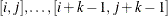in a diagonal direction of thematrix. The special case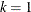can be used to assign values to single nonzero element locations in.

   MATRIX H [1,1]= 4 * 100,
[2,1]= 3 *  10,
[3,1]= 2 *   1;
MATRIX G [1,1]= 1  2  3  4;

• Columnwise Starting in Diagonal: This form of pattern definition is useful if thematrix contains nonzero elements columnwise starting in the diagonal. The starting location is specified by only one indexin brackets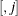. Thenumbers at the right-hand side are assigned to the elements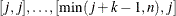.

   MATRIX H [,1]= 100  10   1,
[,2]= 100  10   1,
[,3]= 100  10,
[,4]= 100;
MATRIX G [,1]= 1  2  3  4;

• Rowwise Starting in First Column: This form of pattern definition is useful if thematrix contains nonzero elements rowwise ending in the diagonal. The starting location is specified by only one indexin brackets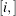. Thenumbers at the right-hand side are assigned to the elements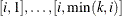.

   MATRIX H [1,]= 100,
[2,]=  10 100,
[3,]=   1  10 100,
[4,]=   0   1  10 100;
MATRIX G [1,]= 1  2  3  4;